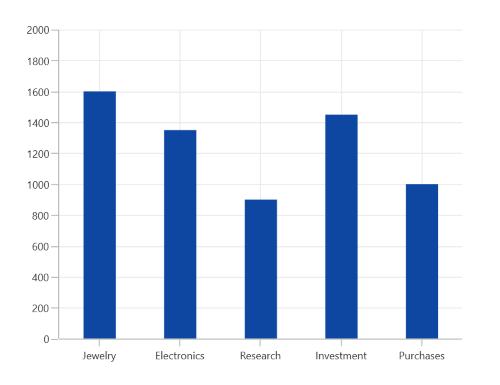# Column Chart in WinUI Chart (SfCartesianChart)

3 Jan 20233 minutes to read

WinUI Column Chart is used to plot discrete rectangles for the given data point values. To render a column chart, create an instance of ColumnSeries, and add it to the Series collection property of SfCartesianChart.

``````<chart:SfCartesianChart>

<chart:SfCartesianChart.XAxes>
<chart:CategoryAxis />
</chart:SfCartesianChart.XAxes>

<chart:SfCartesianChart.YAxes>
<chart:NumericalAxis />
</chart:SfCartesianChart.YAxes>

<chart:SfCartesianChart.Series>
<chart:ColumnSeries ItemsSource="{Binding Data}"
XBindingPath="XValue"
YBindingPath="YValue"/>
</chart:SfCartesianChart.Series>

</chart:SfCartesianChart>``````
``````SfCartesianChart chart = new SfCartesianChart();
CategoryAxis xAxis = new CategoryAxis();
NumericalAxis yAxis = new NumericalAxis();

ColumnSeries series = new ColumnSeries()
{
ItemsSource = new ViewModel().Data,
XBindingPath = "XValue",
YBindingPath = "YValue",
};

this.Content = chart;``````## Segment spacing

The SegmentSpacing property is used to change the spacing between two segments. The default value of spacing is 0, and the value ranges from 0 to 1. Here, 1 and 0 correspond to 100% and 0% of the available space, respectively.

``````<chart:SfCartesianChart>

<chart:SfCartesianChart.XAxes>
<chart:CategoryAxis />
</chart:SfCartesianChart.XAxes>

<chart:SfCartesianChart.YAxes>
<chart:NumericalAxis />
</chart:SfCartesianChart.YAxes>

<chart:SfCartesianChart.Series>
<chart:ColumnSeries ItemsSource="{Binding Data}"
XBindingPath="XValue"
YBindingPath="YValue"
SegmentSpacing="0.5"/>
</chart:SfCartesianChart.Series>

</chart:SfCartesianChart>``````
``````SfCartesianChart chart = new SfCartesianChart();
CategoryAxis xAxis = new CategoryAxis();
NumericalAxis yAxis = new NumericalAxis();

ColumnSeries series = new ColumnSeries()
{
ItemsSource = new ViewModel().Data,
XBindingPath = "XValue",
YBindingPath = "YValue",
SegmentSpacing = 0.5
};